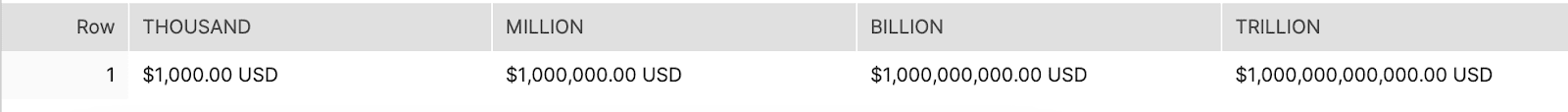# Format Numbers With Comma Separation in Snowflake Result Set

• How-Tos FAQs
• March 29, 2021

In order to customize format numbers with comma separation in Snowflake result set, fields with a number data type first need to be converted to a string, by casting to varchar or char data type. There is an optional SQL format model parameter in number-to-string conversion functions to specify how to format numbers when converting to a string.

To convert a number to text, we use TO_VARCHAR(<numeric_data>) OR TO_CHAR(<numeric_data>) where <numeric_data> is a field with a data type of number. These functions are synonymous, meaning they both do the exact same thing. Using one over the other is a matter of preference.

In order to change the format of the numeric data, we add an additional optional parameter to these functions:

``TO_VARCHAR(<numeric_data>[, <sql_format_model>])``

<sql_format_model> is a string input that determines how a number is displayed when we convert it to text.

Customizing the format of numbers in Snowflake is fairly easy, once you know how SQL Format Models work.

SQL Format Models have two types of components:

• Elements
• Literals

Elements are placeholder characters that are replaced with the number you are formatting or behave in a certain way depending on the length of the number the format model is applied to.

• Numeric elements in Snowflake:
• 0, 9 – replaced with numeric digits
• ,, . – separators
• \$, S, MI – dollar and

Literals are specific characters that are passed through the format model as-is.

• Literals in Snowflake: ., ,, :, ;, , =, /, (, )
• When using literals to format a numeric data type, . and , are treated as elements when following numeric elements 0 and 9
• In addition to the pre-defined literals above, anything wrapped in double quotes will be treated as a literal.

Copy and paste this code if you want to follow along with the examples below. You can replace the format model where commented to see how different format models work on numbers of varying length.

``````with
input as (
select
'9,999,999,999,999' --format model
as format
),
numeric_tests as (
select
1000 as thousand,
thousand * 1000 as million,
million * 1000 as billion,
billion * 1000 as trillion
)

select
to_varchar(thousand, (select format from input)) as thousand,
to_varchar(million, (select format from input)) as million,
to_varchar(billion, (select format from input)) as billion,
to_varchar(trillion, (select format from input)) as trillion

from numeric_tests;``````

Example 1

This first format model adds commas to a number up to 13 digits long. 9 is used as a placeholder for any number, and when no number is in that position, a blank space is returned before the digits so that the length of the string after conversion is still fixed:

``'9,999,999,999,999' --format model``Example 2

If we only specify 10 digits, the numbers longer than 10 digits will overflow and numbers will display as #:

``'9,999,999,999' --format model``

##Example 3

0 can be used instead of 9 to display leading and trailing zeros:

``'9,999,999,000,000.00' --format model``

##Example 4

Add a dollar sign with the \$ element:

``'\$9,999,999,999,999.00' --format model``

##Example 5

Add a custom literal using double quotes:

``'\$9,999,999,999,999.00 "USD"' --format model``As you can see, SQL Format Models are a very useful tool for creating formatted reports in SQL. You can utilize this transformation technique in Datameer using the SQL Editor transformation.

Adding comma separation in Snowflake is pretty straightforward once you understand how SQL Format Models work in Snowflake. The examples above cover the most common use cases to format numbers with comma separation in Snowflake result set. Snowflake goes into more detail and has additional use cases for SQL Format Models in Snowflake’s documentation.